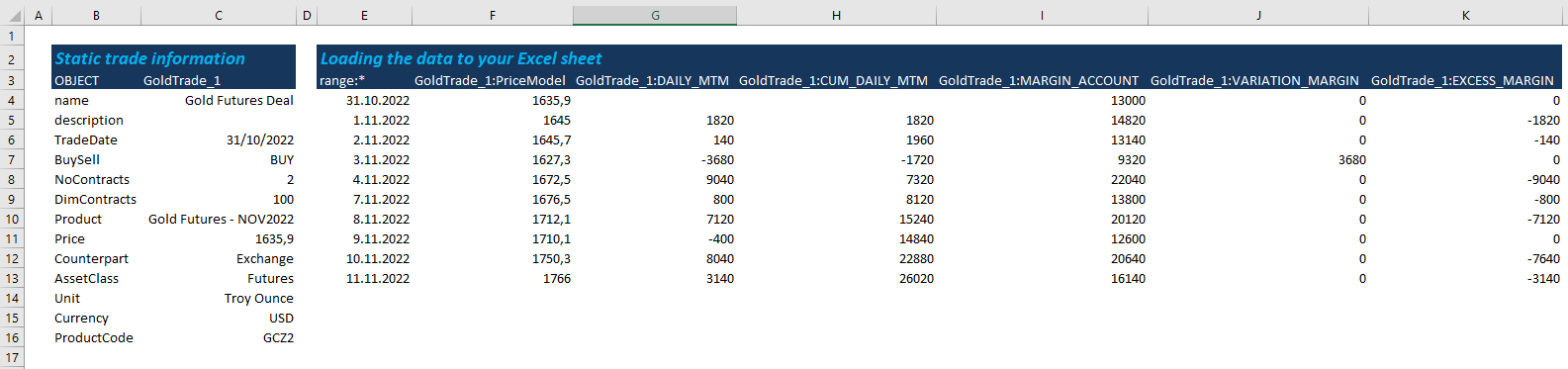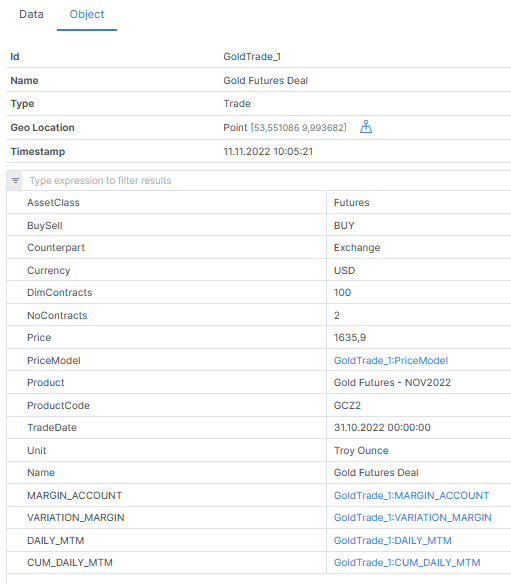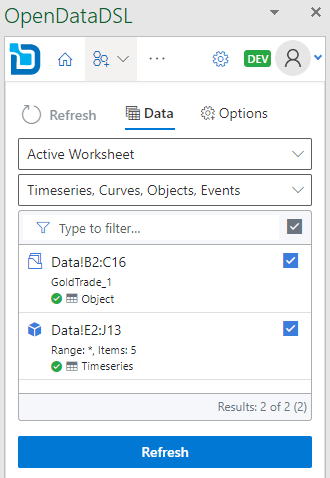# Margin account calculations in ODSL## Margin account calculations in ODSL?

Explore for a Gold futures deal margin account what the inputs are, what calculations are required and which data are stored and where.

## Gold futures deal margin account​

### Inputs​

If you run the calculation in Excel, your sheet might look similar to the following with the daily marking-to-market for your position of 200 troy ounces gold futures November contract. At the top you find the trade information and at the bottom the margin account calculations.Besides specific trade information, an updating price series needs to be defined to run the daily marking to market of the gold futures trade. For this example we use gold futures settlements from the CME website, where usually settlement prices of the last 5 business days are listed. We loaded the data for testing purpose using the ODSL Excel Addin.

The CME website provides some spec as well as margins information.

### Margin calculations​

The initial margin is the amount that must be deposited when the contract is entered, here we have 6,500 USD per contract or 13,000 USD in total. At the end of each trading day the margin account is updated to reflect the gain or loss - basically the daily marking to market.

A maintenance margin is set to make sure the margin account balance never becomes negative, here we use 5,700 USD per contract or 11,400 USD in total. In case the balance of the margin account is below the maintenance margin, a margin call is triggered. The variation margin is the amount to be placed to the margin account up to the target of the initial margin.

Additionally, we calculate the excess margin that can be withdrawn from the margin account. The excess margin is the amount above the total initial margin.

### Automate the calculation process with smart time series​

For the business logic create an ODSL script (here we call it margin-functions.odsl) in Microsoft VSCode for easy coding using our DSL (4GL language) to calculate

• (cumulated) daily MTM,
• margin account,
• variation margin,
• excess margin

stored as smart time series.

Smart time series

Why smart time series? Because an input feed (BASE input) triggers the calculation and inherits the calendar information (business with specific holidays) to the dependent time series. We think this is smart.

An example for the margin account function using the data management specific syntax:

``//define a function with specific input parametersfunction marginAccount(price, initialMargin, maintenanceMargin, ncontracts, contract)    /*    mtm: daily marking-to-market; ODSL function diff is called for daily price change    start: start for the time series    result: time series storing the margin account with:         initial value = initial margin for contracts and size     margin: total initial margin    variationMargin: initial value of the variation margin    excessMargin: initial value of the excess margin    */    mtm = diff(price)*ncontracts*contract    start = mtm.calendar.previous(mtm.start)    result = TimeSeries(start, "business", initialMargin * ncontracts)    margin = initialMargin * ncontracts    variationMargin = 0    excessMargin = 0    /*    Calculating the values for day-to-day margin account, variation and excess margin:    margin account on day t is:     daily MtM from t + (margin account value + variation margin + excess margin) from t-1    variation margin is:     positive when the value of the margin account is below the maintenance margin     and filled up to the initial margin    excess margin is:    negative when the value of the margin account is above initial margin    the value above initial margin is withdrawn from the margin account    */    for tv in mtm.values        margin = margin + tv.value + variationMargin + excessMargin        result.addValue(margin)        if margin < maintenanceMargin * ncontracts            variationMargin = initialMargin * ncontracts - margin            excessMargin = 0        elseif margin > initialMargin * ncontracts            excessMargin = initialMargin * ncontracts - margin            variationMargin = 0        else             variationMargin = 0            excessMargin = 0        end    next    marginAccount=resultend``

### Easily configure the smart time series directly in the WebPortal​

Input price data from any datasource - we chose CME data for this example - trigger the calculation process for (cumulated) daily MTM, margin account, variation margin and excess margin. Moreover, as soon as the daily MTM got calculated, it triggers the cumulated daily MTM - simply smart.

Select the appropriate script margin-functions[.odsl] from the list, call the functions with specific input data according to the Excel sheet.

In this example, it is marginAccount(BASE, 6,500, 5,700, 2, 100) whereas BASE is the price input for daily marking-to-market. The initial margin is 6,500 USD per contract x contract size, the maintenance margin is 5,700 USD per contract x contract size, having a contract size of 100 and 2 contracts in total.

Run a test and save the configuration with just a click.### Analyse the output and setup in the WebPortal​The object collects all static data as well as the time series data.### ...or load them into an Excel Sheet​

Pull the static trade and time series data into an Excel Sheet